# need help ASAP with 6 and 7. Thank You! (6)(20 points) (a) Let G be a...

need help ASAP with 6 and 7. Thank You!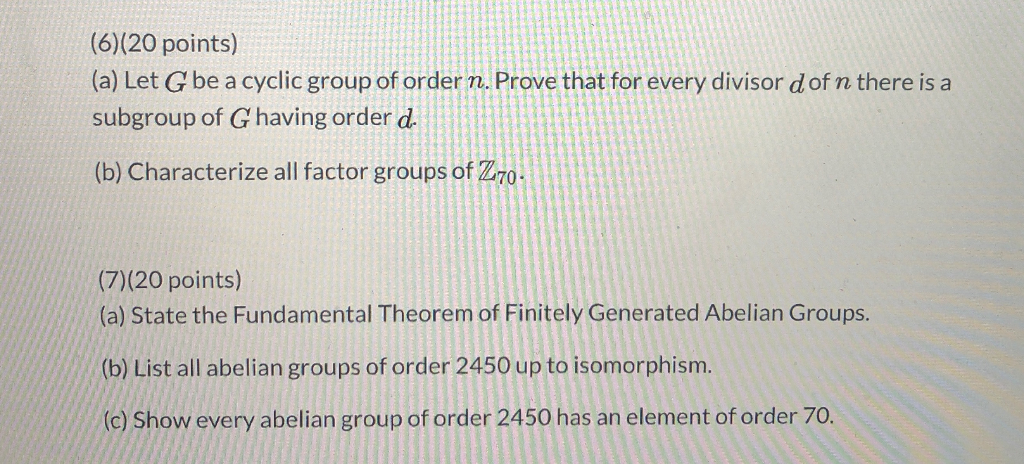(6)(20 points) (a) Let G be a cyclic group of order n. Prove that for every divisor dof n there is a subgroup of Ghaving order d. (b) Characterize all factor groups of Z70. (7)(20 points) (a) State the Fundamental Theorem of Finitely Generated Abelian Groups. (b) List all abelian groups of order 2450 up to isomorphism. (c) Show every abelian group of order 2450 has an element of order 70.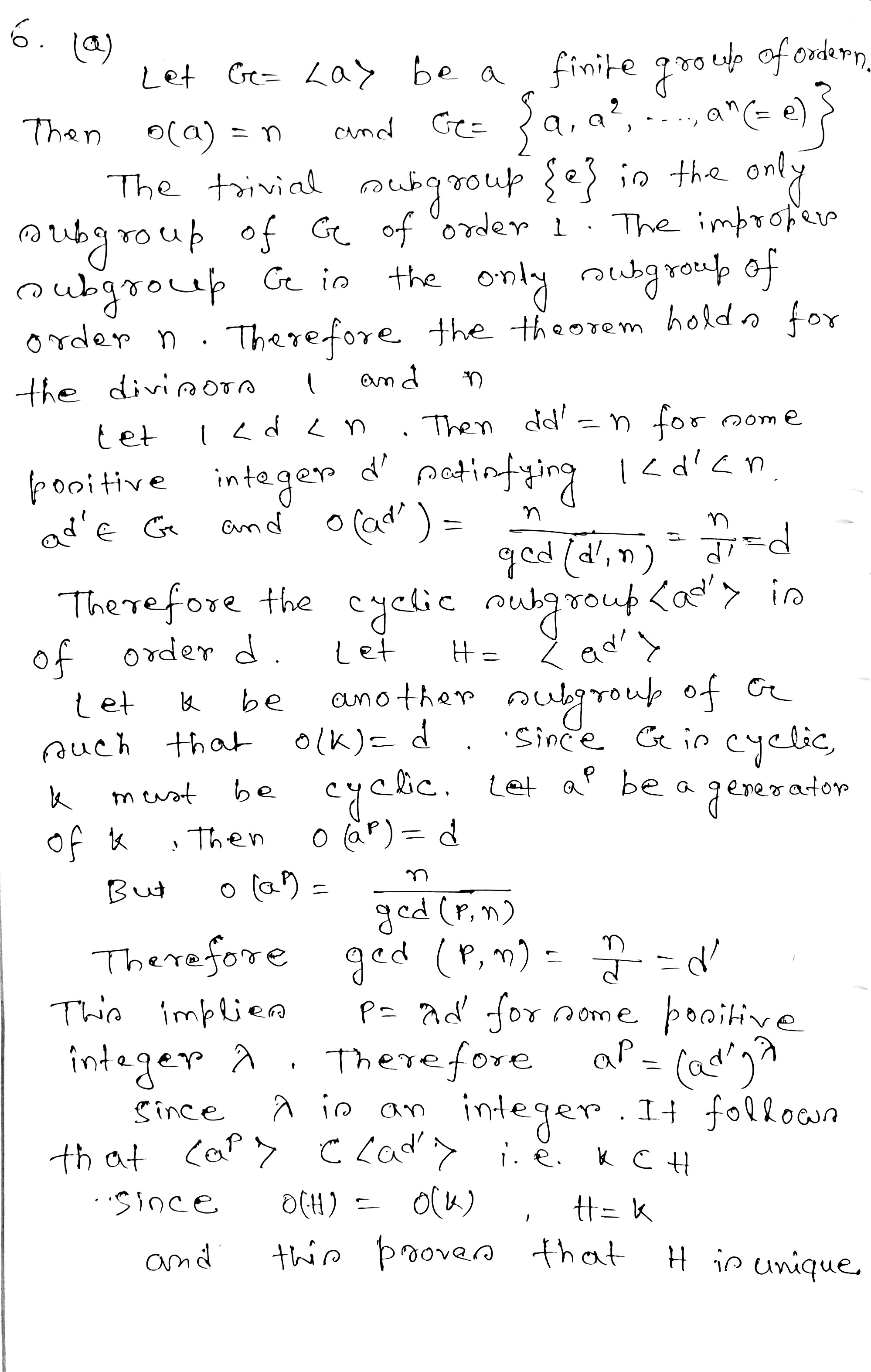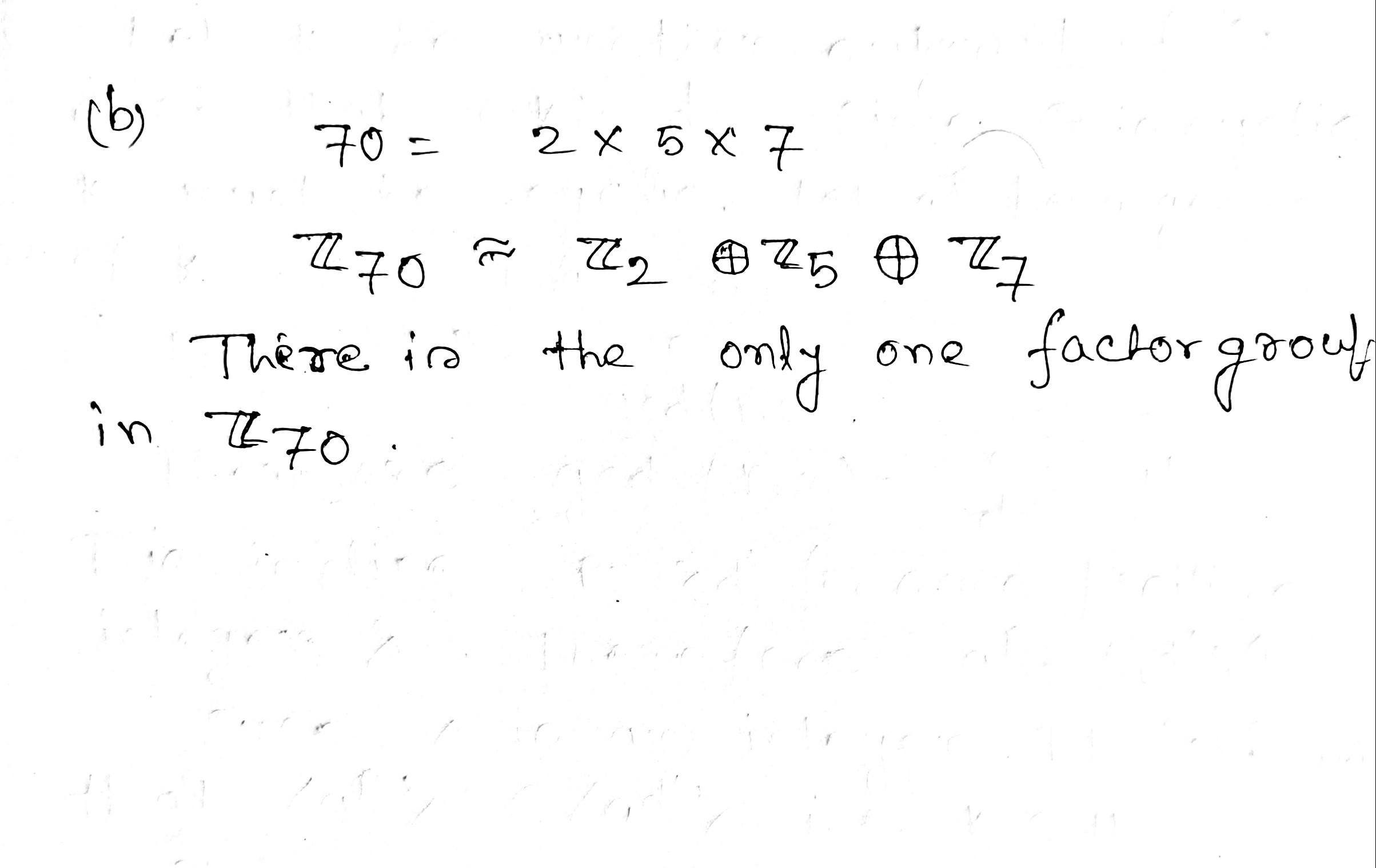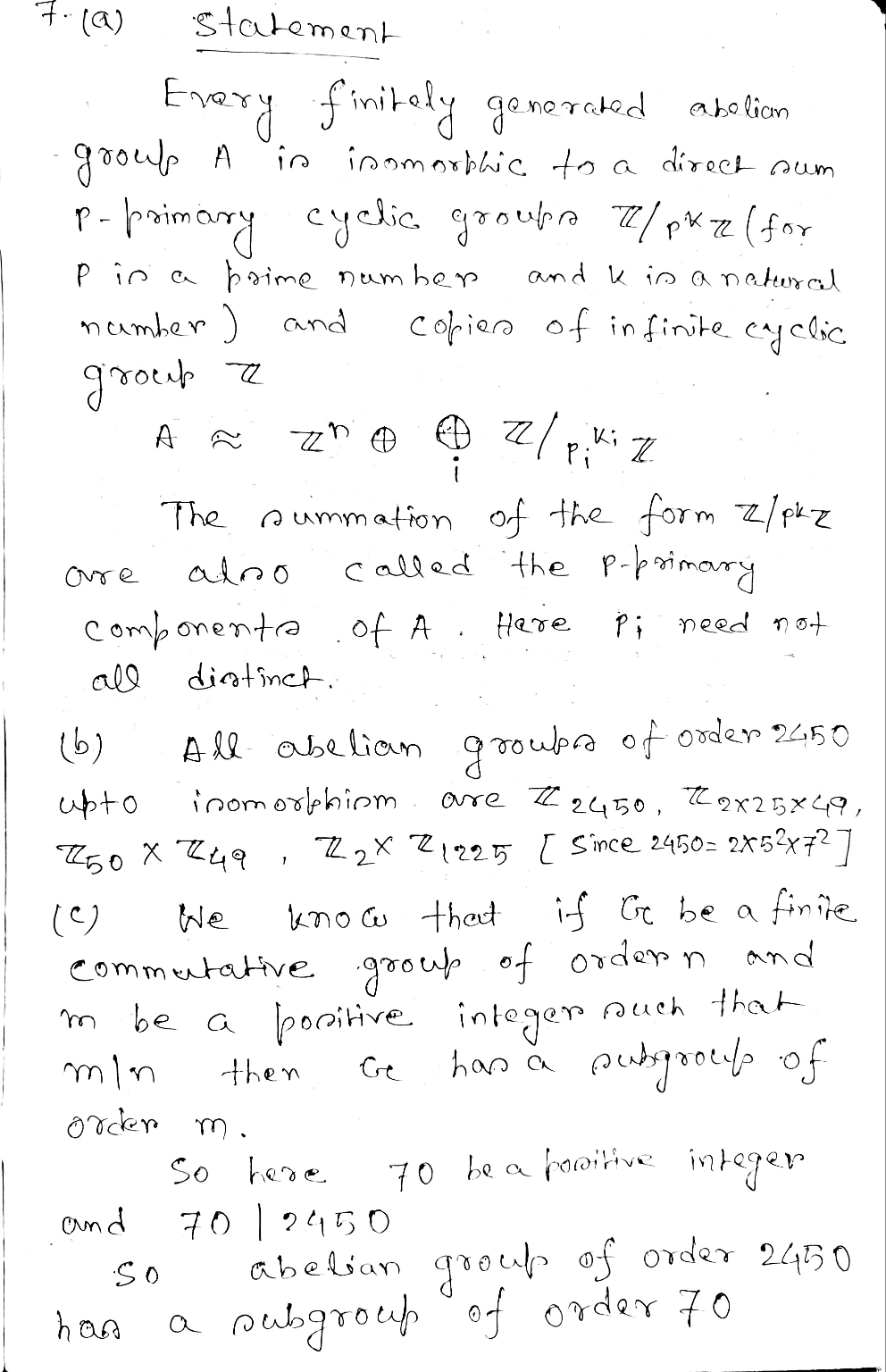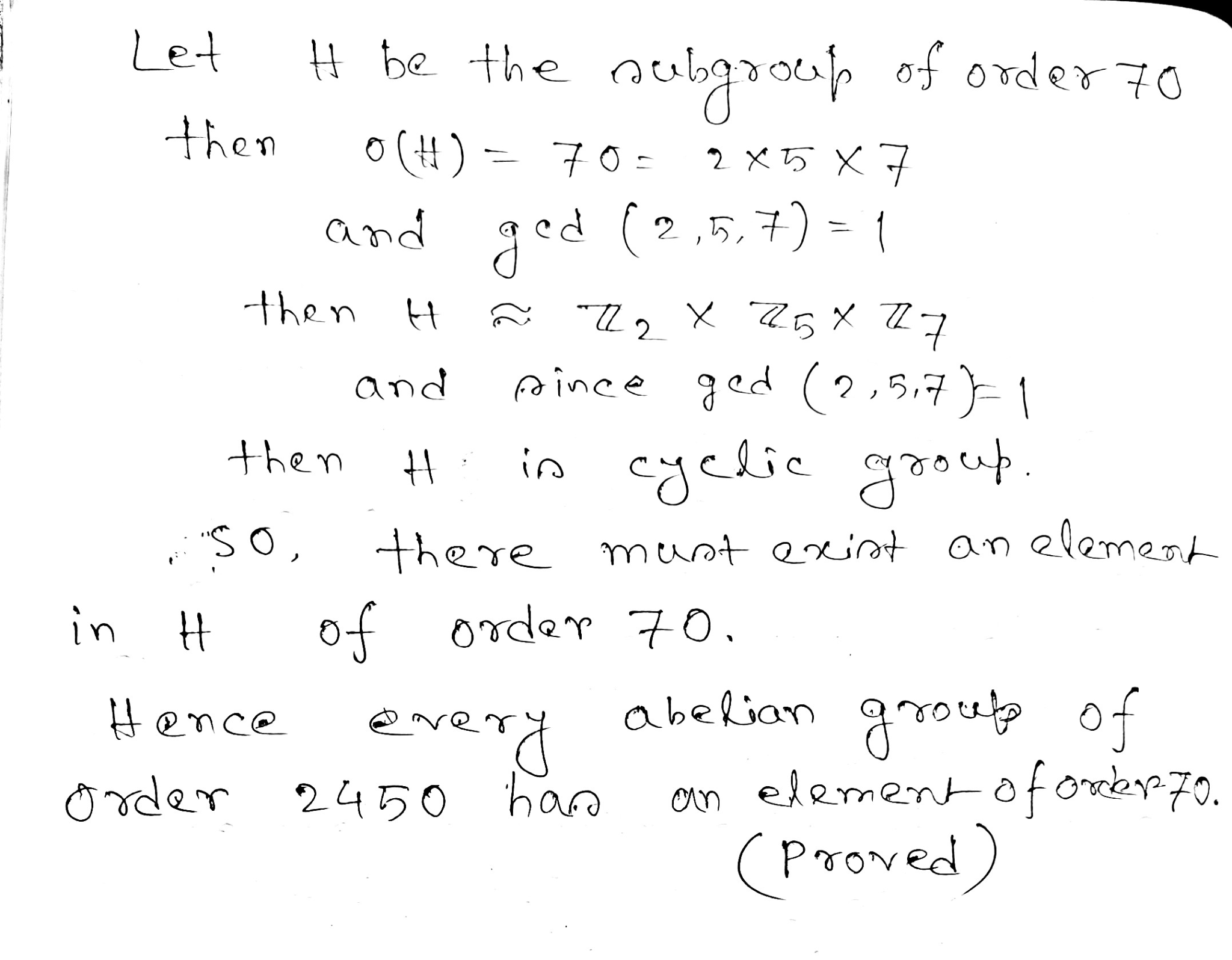##### Add Answer of: need help ASAP with 6 and 7. Thank You! (6)(20 points) (a) Let G be a...
Similar Homework Help Questions
• ### ASAP PLEASE (6)(20 points) (a) Let G be a cyclic group of order n. Prove that...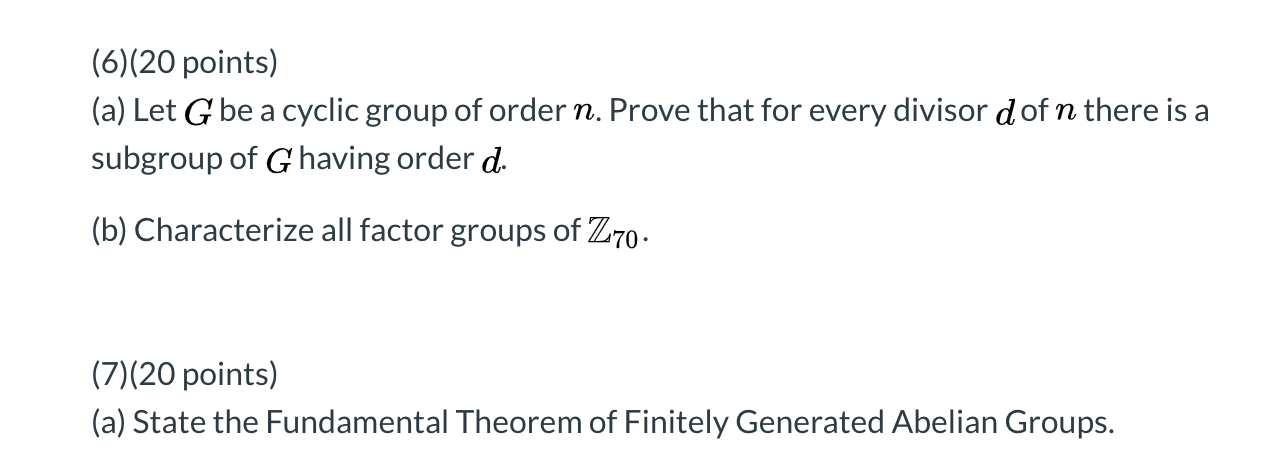ASAP PLEASE (6)(20 points) (a) Let G be a cyclic group of order n. Prove that for every divisor dof n there is a subgroup of G having order d. (b) Characterize all factor groups of Z70. (7)(20 points) (a) State the Fundamental Theorem of Finitely Generated Abelian Groups.

• ### (5)(20 points) In the problems below, give the order of the element in the indicated factor...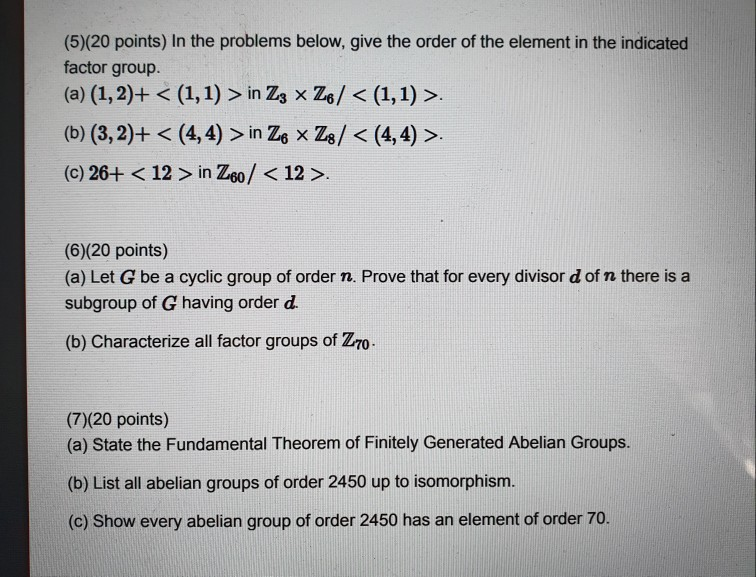(5)(20 points) In the problems below, give the order of the element in the indicated factor group. (a) (1, 2)+ < (1,1) > in Z3 x Z6/ < (1,1) >. (b) (3,2)+ < (4,4) > in Ze * Z8/ < (4,4) >. (c) 26+ < 12 > in Z60 / <12>. (6)(20 points) (a) Let G be a cyclic group of order n. Prove that for every divisor d of n there is a subgroup of G having order d....

• ### (7)(20 points) (a) State the Fundamental Theorem of Finitely Generated Abelian Groups. (b) List all abelian...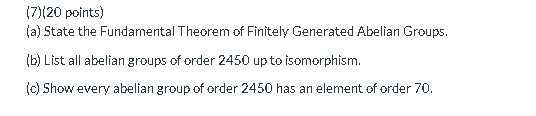(7)(20 points) (a) State the Fundamental Theorem of Finitely Generated Abelian Groups. (b) List all abelian groups of order 2450 up to isomorphism. (c) Show every abelian group of order 2450 has an element of order 70.

• ### (7)(20 points) (a) State the Fundamental Theorem of Finitely Generated Abelian Groups. (b) List all abelian...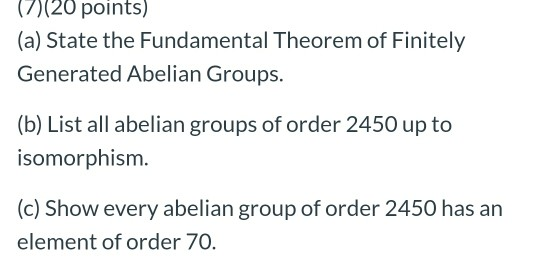(7)(20 points) (a) State the Fundamental Theorem of Finitely Generated Abelian Groups. (b) List all abelian groups of order 2450 up to isomorphism. (c) Show every abelian group of order 2450 has an element of order 70.

• ### (6)(20 points) (a) Let G be a cyclic group of order n. Prove that for every...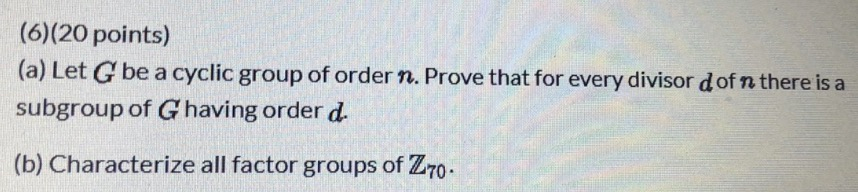(6)(20 points) (a) Let G be a cyclic group of order n. Prove that for every divisor dofn there is a subgroup of Ghaving order d. (b) Characterize all factor groups of Z70.

• ### (6)(20 points) (a) Let G be a cyclic group of order n. Prove that for every...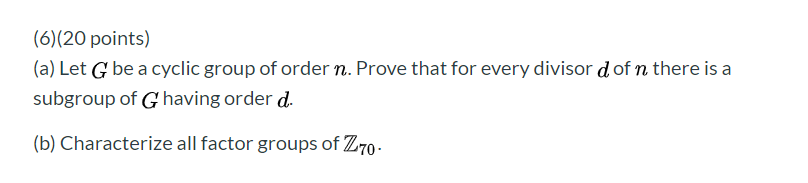(6)(20 points) (a) Let G be a cyclic group of order n. Prove that for every divisor d of n there is a subgroup of G having order d. (b) Characterize all factor groups of Z70 -

• ### (a) State the Fundamental Theorem of Finitely Generated Abelian Groups. (b) List all abelian groups of...

(a) State the Fundamental Theorem of Finitely Generated Abelian Groups. (b) List all abelian groups of order 2450 up to isomorphism. (c) Show every abelian group of order 2450 has an element of order 70.

• ### 8. (20 points) Let G Zs x Zg and let H be the cyclic subgroup generated by (3, 3). (a) Find the o...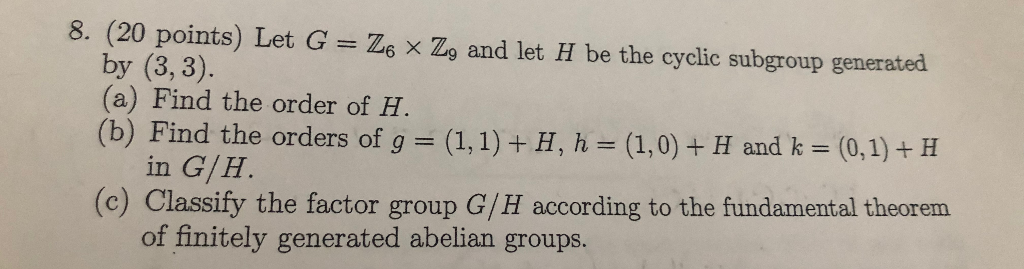8. (20 points) Let G Zs x Zg and let H be the cyclic subgroup generated by (3, 3). (a) Find the order of H (b) Find the orders of g = (1,1) + H, h = (1,0) + H and k = (0,1) + H in G/H (c) Classify the factor group G/H according to the fundamental theorem of finitely generated abelian groups. 8. (20 points) Let G Zs x Zg and let H be the cyclic subgroup generated...

• ### (6)(20 points) (a) Let G be a cyclic group of order 72. Prove that for every...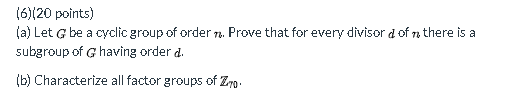(6)(20 points) (a) Let G be a cyclic group of order 72. Prove that for every divisor d of a there is a subgroup of G having order d. (b) Characterize all factor groups of Zyo.

• ### (a) Let G be a cyclic group of order n. Prove that fo every divisor d...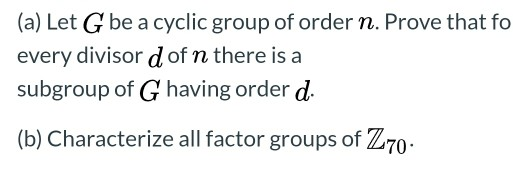(a) Let G be a cyclic group of order n. Prove that fo every divisor d of n there is a subgroup of G having order d. (b) Characterize all factor groups of Z70.

Free Homework App